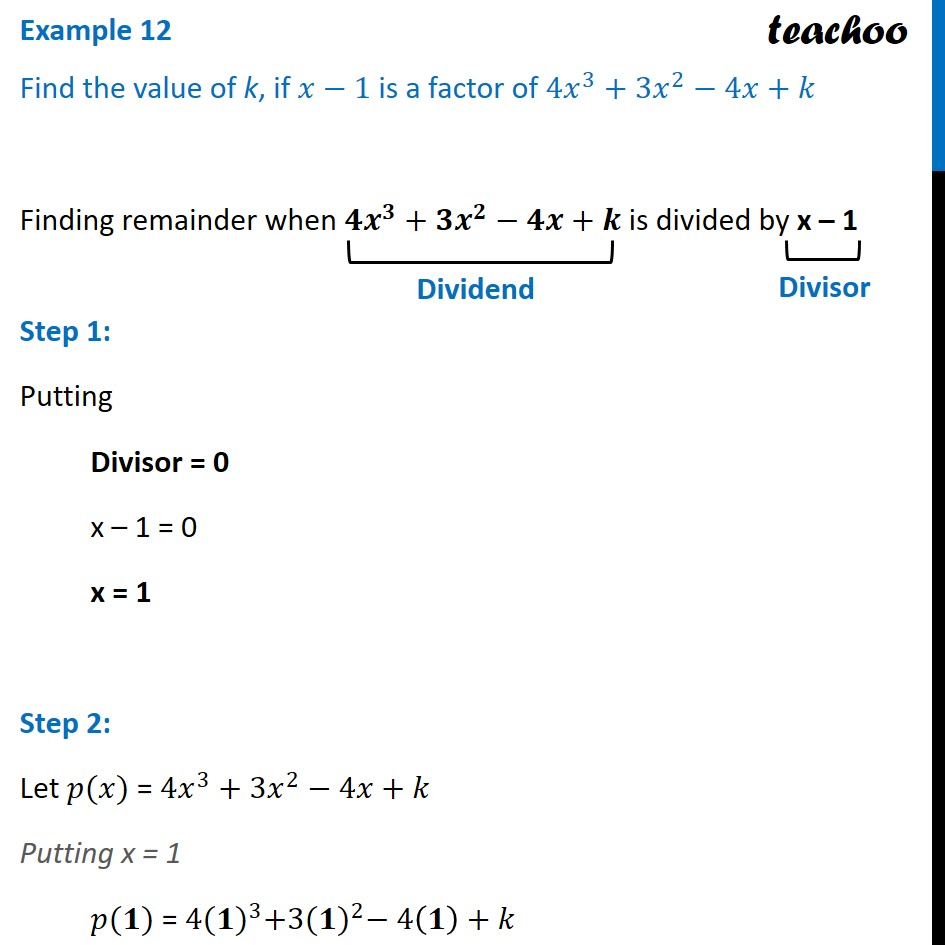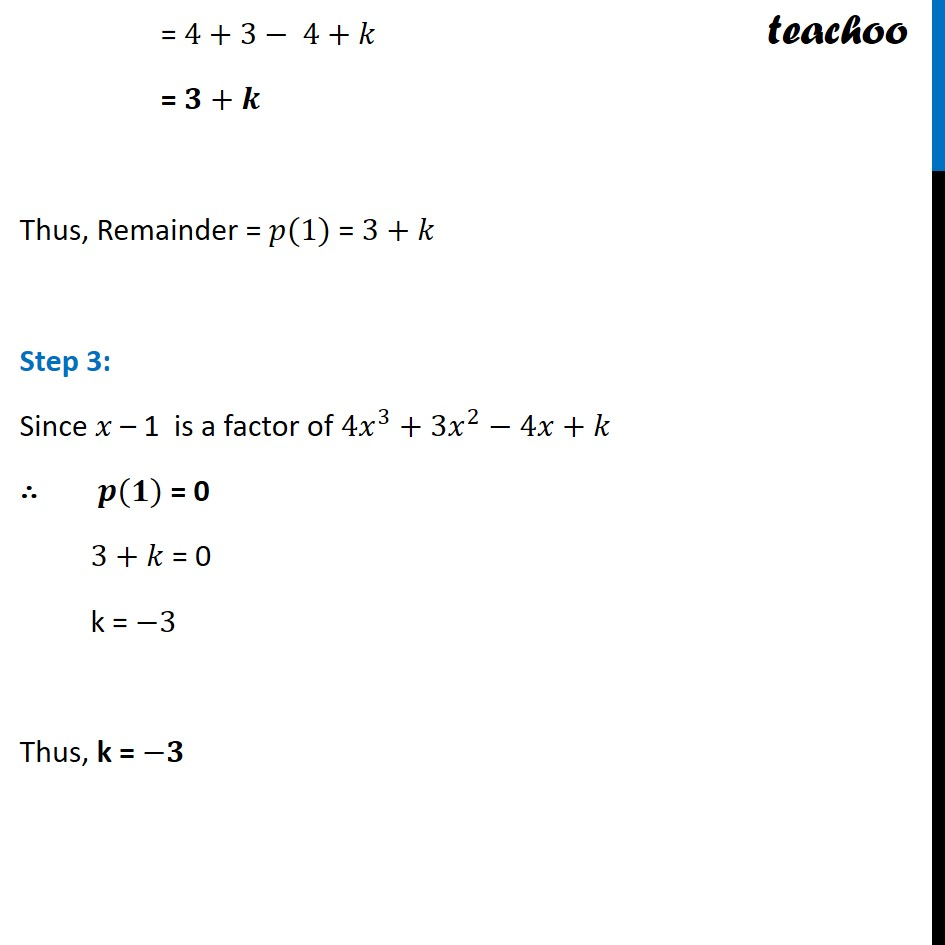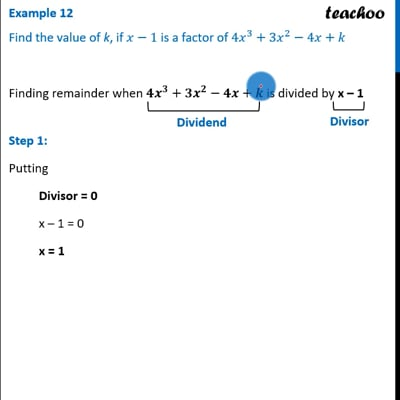Examples

Chapter 2 Class 9 Polynomials
Serial order wiseThis video is only available for Teachoo black users

Solve all your doubts with Teachoo Black (new monthly pack available now!)

### Transcript

Example 12 Find the value of k, if 𝑥−1 is a factor of 4𝑥^3+3𝑥^2−4𝑥+𝑘 Finding remainder when 𝟒𝒙^𝟑+𝟑𝒙^𝟐−𝟒𝒙+𝒌 is divided by x – 1 Step 1: Putting Divisor = 0 x – 1 = 0 x = 1 Step 2: Let 𝑝(𝑥) = 4𝑥^3+3𝑥^2−4𝑥+𝑘 Putting x = 1 𝑝(𝟏) = 4〖(𝟏)〗^3+3〖(𝟏)〗^2− 4(𝟏)+𝑘 Dividend Divisor = 4+3− 4+𝑘 = 𝟑+𝒌 Thus, Remainder = 𝑝(1) = 3+𝑘 Step 3: Since 𝑥 – 1 is a factor of 4𝑥^3+3𝑥^2−4𝑥+𝑘 ∴ 𝒑(𝟏) = 0 3+𝑘 = 0 k = −3 Thus, k = −𝟑